## Sphere with Tunnel

Find the tunnel between two pointsandon a gravitating Sphere which gives the shortest transit time under the force of gravity.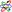Assume the Sphere to be nonrotating, of Radius, and with uniform density. Then the standard form Euler-Lagrange Differential Equation in polar coordinates is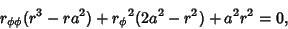(1)

along with the boundary conditions,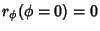,, and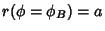. Integrating once gives(2)

But this is the equation of a Hypocycloid generated by a Circle of Radiusrolling inside the Circle of Radius, so the tunnel is shaped like an arc of a Hypocycloid. The transit time from pointto pointis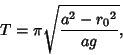(3)

where(4)

is the surface gravity with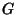the universal gravitational constant.